2023-5-26 22:32
10403

## 时间线

2023-02-27：发现漏洞，并将该漏洞上报给了厂商
2023-02-28：厂商确认了漏洞，并启动了应急响应，开始修补并内测
2023-03-06：厂商给予了一定的奖励（不过有点少QAQ）
2023-05-24：厂商修复了所有影响的设备并发布了安全通告及致谢

CVSS 3.1评分：9.8（严重，CVSS:3.1/AV:N/AC:L/PR:N/UI:N/S:U/C:H/I:H/A:H）

## 固件解密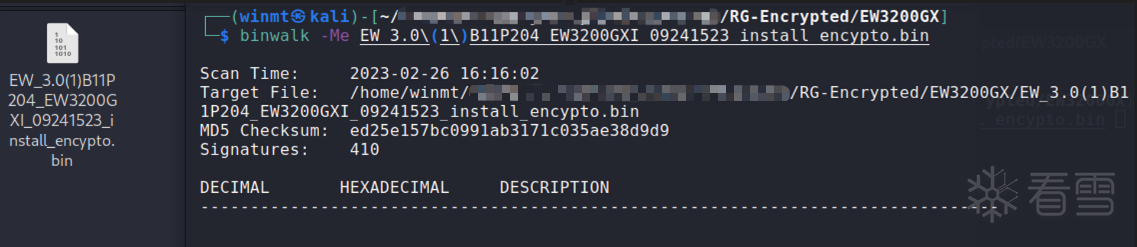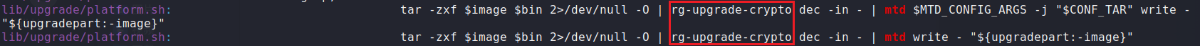（由于该加解密算法仍然被广泛应用于某捷的各类核心产品中，故这里不放出具体逆向分析的过程，此处省略xxx字........）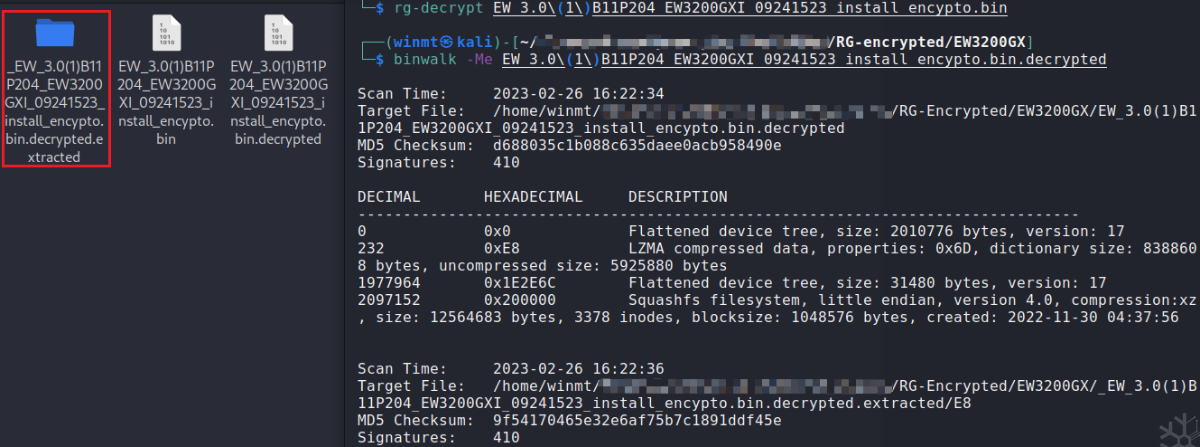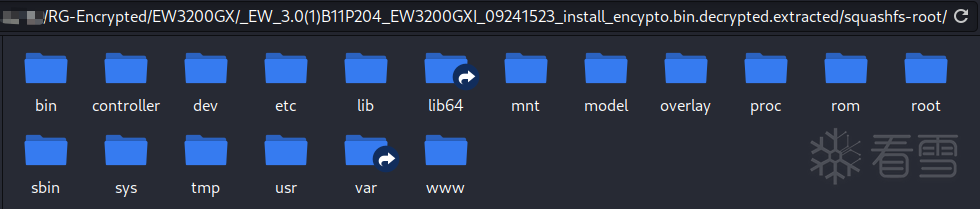## 漏洞分析

### 找到无鉴权的API接口

 1 `entry({``"api"``, ``"auth"``}, call(``"rpc_auth"``), nil).sysauth ``=` `false`

 123456 `function rpc_auth()``    ``...``    ``local _tbl ``=` `require ``"luci.modules.noauth"``    ``...``    ``ltn12.pump.``all``(jsonrpc.handle(_tbl, http.source()), http.write)``end`

### 寻找可能存在漏洞的入口

`noauth.lua`中，有`login``singleLogin``merge``checkNet`四个方法。其中，`singleLogin`函数无可控制的参数，不用看；`checkNet`函数中参数可控的字段只有`params.host`，并拼接入了命令字符串执行，但是在之前有`tool.checkIp(params.host)`对其的合法性进行了检查，无法绕过。

 12345678910111213141516 `function login(params)``    ``...``    ``if` `params.password ``and` `tool.includeXxs(params.password) then``        ``tool.eweblog(``"INVALID DATA"``, ``"LOGIN FAILED"``)``        ``return``    ``end``    ``...``    ``local checkStat ``=` `{``        ``password ``=` `params.password,``        ``username ``=` `"admin"``, ``-``-` `params.username,``        ``encry ``=` `params.encry,``        ``limit ``=` `params.limit``    ``}``    ``local authres, reason ``=` `tool.checkPasswd(checkStat)``    ``...``end`

 1234567891011 `function checkPasswd(checkStat)``    ``...``    ``local _data ``=` `{``        ``type` `=` `checkStat.encry ``and` `"enc"` `or` `"noenc"``,``        ``password ``=` `checkStat.password,``        ``name ``=` `checkStat.username,``        ``limit ``=` `checkStat.limit ``and` `"true"` `or` `nil``    ``}``    ``local _check ``=` `cmd.devSta.get({module ``=` `"adminCheck"``, device ``=` `"pc"``, data ``=` `_data})``    ``...``end`

 1234 `function includeXxs(``str``)``    ``local ngstr ``=` `"[`&\$;|]"``    ``return` `string.match(``str``, ngstr) ~``=` `nil``end`

 123456 `devSta[opt[i]] ``=` `function(params)``local model ``=` `require ``"dev_sta"``params.method ``=` `opt[i]``params.cfg_cmd ``=` `"dev_sta"``local data, back, ip, password, shell ``=` `doParams(params)``return` `fetch(model.fetch, shell, params, opt[i], params.module, data, back, ip, password)`

 1234 `if` `params.data then``    ``data ``=` `luci.json.encode(params.data)``    ``_shell ``=` `_shell .. ``" '"` `.. data .. ``"'"``end`

 1234 `function merge(params)``    ``local cmd ``=` `require ``"luci.modules.cmd"``    ``return` `cmd.devSta.``set``({device ``=` `"pc"``, module ``=` `"networkId_merge"``, data ``=` `params, async ``=` `true})``end`

### 进一步分析参数传递过程

`noauth.lua``merge`函数中，调用了`devSta.set`函数，同样是对应着`cmd.lua`中的如下位置，此时`opt[i]`循环到了`set`方式。此时，由于之前没有任何过滤，无需使用换行符作为命令分隔符，最简单的分号、反引号之类的即可，故`doParams`函数中的`encode`不会造成影响。

 123456 `devSta[opt[i]] ``=` `function(params)``local model ``=` `require ``"dev_sta"``params.method ``=` `opt[i]``params.cfg_cmd ``=` `"dev_sta"``local data, back, ip, password, shell ``=` `doParams(params)``return` `fetch(model.fetch, shell, params, opt[i], params.module, data, back, ip, password)`

 12345 `local function fetch(fn, shell, params, ...)``    ``...``    ``local _res ``=` `fn(...)``    ``...``end`

`/usr/lib/lua/dev_sta.lua``fetch`函数中，这里的`cmd``set`方式，`module``networkId_merge`，而此处的`param`就是我们可控的`data`字段（即最初`POST`报文中`params`的内容）。可见，对一些字段赋予了真假值后，最终将参数都传递给了`/usr/lib/lua/libuflua.so`中的`client_call`函数。接下来，就是对二进制文件逆向分析并寻找是否存在命令执行点了。

 123456 `function fetch(cmd, module, param, back, ip, password, force, not_change_configId, multi)``    ``local uf_call ``=` `require ``"libuflua"``    ``...``    ``local stat ``=` `uf_call.client_call(ctype, cmd, module, param, back, ip, password, force, not_change_configId, multi)``    ``...``end`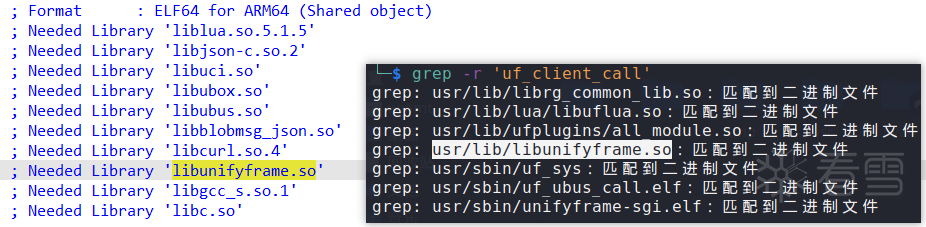`/usr/lib/libunifyframe.so``uf_client_call`函数中，先将传入的`data`等字段转为`Json`格式的数据，作为`param`字段的内容。然后将`Json`数据通过`uf_socket_msg_write``socket`套接字（分析可知，此处采用的是本地通信的方式）进行数据传输

 12345678910 `      ``json_object_object_add(v22, ``"data"``, v35);``LABEL_82:``      ``...``      ``json_object_object_add(v5, ``"params"``, v22);``      ``v44 ``=` `(const char ``*``)json_object_to_json_string(v5);``      ``...``      ``v45 ``=` `uf_socket_client_init(``0LL``);``      ``...``      ``v51 ``=` `strlen(v44);``      ``uf_socket_msg_write(v45, v44, v51);`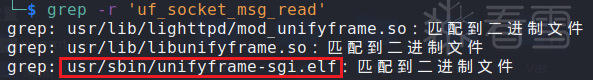123456789101112131415161718192021 `\$ cat etc``/``init.d``/``unifyframe``-``sgi` `...``PROG``=``/``usr``/``sbin``/``unifyframe``-``sgi.elf``...` `if` `[ ``-``f ``"\$IPKG_INSTROOT/lib/functions/procd.sh"` `]; then``    ``...``else``    ``...``    ``start() {``        ``...``        ``echo ``"Starting \$PROG ..."``        ``service_start \$PROG``        ``...``    ``}` `    ``stop() {``        ``...``    ``}``fi`

### 逆向分析并寻找命令执行点

`unifyframe-sgi.elf`中，`uf_socket_msg_read`函数交叉引用，找到`socket`数据接收点。如下方代码段，`v6 = 0x432000`，简单计算一下，可知`v57`即为`uf_socket_msg_read`函数，其中接收到的数据存储在`v56`。接收到的`Json`数据形如`{"method":"devSta.set", "params":{"module":"networkId_merge", "async":true, "data":"xxx"}}`（可结合上文，自行分析得出），其中`data`字段可控。

 123456 `v6 ``=` `0x432000uLL``;``...``v57 ``=` `*``(__int64 (__fastcall ``*``*``)(__int64, unsigned ``int` `*``*``))(v6 ``+` `1784``); ``/``/` `uf_socket_msg_read``v58 ``=` `*``v46;``*``v56 ``=` `v46;``v59 ``=` `v57(v58, v56 ``+` `1``);`

 123456 `if` `( !(``*``(unsigned ``int` `(__fastcall ``*``*``)(unsigned ``int` `*``*``))(v6 ``+` `3328``))(v56) ) ``/``/` `0x432D00` `parse_content``{``    ``...``    ``if` `( !(``*``(unsigned ``int` `(__fastcall ``*``*``)(unsigned ``int` `*``*``))(v6 ``+` `1776``))(v56) ) ``/``/` `0x4326F0` `add_pkg_cmd2_task``    ``...``}`

 12345678910111213141516171819202122 `if` `( (unsigned ``int``)json_object_object_get_ex(v4, ``"method"``, &v15) ``=``=` `1` `)``{``    ``v6 ``=` `(const char ``*``)json_object_get_string(v15);``    ``...``    ``if` `( strstr(v6, ``"cmdArr"``) )``    ``{``        ``...``    ``}``    ``else``    ``{``        ``*``(_DWORD ``*``)(a1 ``+` `60``) ``=` `1``;``        ``v13 ``=` `malloc_cmd();``        ``if` `( v13 )``        ``{``            ``v14 ``=` `parse_obj2_cmd(v4, string);``            ``...``        ``}``        ``else``        ``{``            ``...``        ``}``    ``}`

`parse_obj2_cmd`函数中，需要注意记录一下各字段存储位置的偏移，后续逆向过程需要用到。这里暂且只记录两个，`from_url`字段的偏移为`81`，我们可控的`data`字段的偏移为`24``v5``QWORD`类型，八字节）。

 12345678910 `if` `( (unsigned ``int``)json_object_object_get_ex(v42, ``"from_url"``, &v43) ``=``=` `1` `)``{``    ``v21 ``=` `(const char ``*``)sub_4069B8(v43);``    ``v22 ``=` `(char ``*``)v21;``    ``if` `( v21 )``    ``{``        ``if` `( ``*``v21 ``=``=` `49` `|| !strcmp(v21, ``"true"``) )``            ``*``((_BYTE ``*``)v5 ``+` `81``) ``=` `1``;``        ``free(v22);``    ``}`
 123456789101112 `_QWORD ``*``v5; ``/``/` `x20``...``if` `( (unsigned ``int``)json_object_object_get_ex(v42, ``"data"``, &v43) ``=``=` `1``    ``&& (unsigned ``int``)json_object_get_type(v43) ``-` `4` `<``=` `2` `)``{``    ``if` `( json_object_get_string(v43) )``    ``{``        ``v31 ``=` `((__int64 (``*``)(void))strdup)();``        ``v5[``3``] ``=` `v31;``        ``...``    ``}``}`

 1234567891011121314 `if` `( (unsigned ``int``)json_object_object_get_ex(v42, ``"async"``, &v43) ``=``=` `1` `)``{``    ``v15 ``=` `(const char ``*``)sub_4069B8(v43);``    ``v16 ``=` `(char ``*``)v15;``    ``if` `( v15 )``    ``{``        ``if` `( ``*``v15 ``=``=` `48` `|| !strcmp(v15, ``"false"``) )``        ``{``            ``*``((_BYTE ``*``)v5 ``+` `76``) ``=` `1``;``            ``*``((_BYTE ``*``)v5 ``+` `77``) ``=` `1``;``        ``}``        ``...``    ``}``}`

 1234 `if` `( (unsigned ``int``)uf_cmd_call(``*``v4, v4 ``+` `1``) )``    ``v13 ``=` `2``;``else``    ``v13 ``=` `1``;`

`uf_cmd_call`函数中，乍一看，貌似有一个命令执行点，这里的`v20`是偏移`24`的位置，也就是`data`字段内容，之后将`data`字段中的数据转成`Json`格式存入`v24`，然后从中提取`url`字段的内容拼接入命令字符串中，并用`popen`执行。

 1234567891011121314 `v20 ``=` `*``((_QWORD ``*``)a1 ``+` `3``);``...``v24 ``=` `json_tokener_parse(v20);``...``v26 ``=` `json_object_object_get(v24, ``"url"``);``v27 ``=` `v26;``...``v28 ``=` `(const char ``*``)json_object_get_string(v27);``...``v33 ``=` `snprintf(v30, v32 ``+` `127``, ``"curl -m 5 -s -k -X GET \"%s"``, v28);``...``while` `( ``1` `)``{``    ``ufm_popen(v30, v84);`

 1234567 `v19 ``=` `*``((unsigned __int8 ``*``)a1 ``+` `81``);``...``if` `( !v19 )``{``    ``v85[``19``] ``=` `*``((_QWORD ``*``)a1 ``+` `3``);``    ``goto LABEL_96;``}`

`LABEL_96`处开始是一堆字段的提取，存放入`v85`数组中，还有一些关于`v85`数组中数据的处理。这里需要关注的是：`v85`偏移`8``9`的位置为`a1`偏移`77``76`的位置（上文分析过，此时这两个偏移的值均为`0`

 12 `LOBYTE(v85[``1``]) ``=` `*``((_BYTE ``*``)a1 ``+` `77``);``BYTE1(v85[``1``]) ``=` `*``((_BYTE ``*``)a1 ``+` `76``);`

 123 `v8 ``=` `ufm_handle(v85);``pthread_mutex_unlock((pthread_mutex_t ``*``)(v85[``23``] ``+` `152``));``pthread_cleanup_pop(v84, ``0LL``);`

`ufm_handle`函数中，由于我们是`set`方式，因此会调用到`sub_410140`函数。

 123456789 `if` `( strcmp((const char ``*``)v6, ``"get"``) )``{``    ``v1 ``=` `"uniframe_sgi/error.log"``;``    ``if` `( !strcmp((const char ``*``)v6, ``"set"``)``        ``|| !strcmp((const char ``*``)v6, ``"add"``)``        ``|| !strcmp((const char ``*``)v6, ``"del"``)``        ``|| !strcmp((const char ``*``)v6, ``"update"``) )``    ``{``        ``v33 ``=` `sub_410140(v3);`

 123 `v6 ``=` `json_object_object_get(a1[``22``], ``"sn"``);``if` `( !v6 )``    ``goto LABEL_36;`

 123 `LABEL_36:``  ``...``  ``v5 ``=` `sub_40DA38(a1, a1 ``+` `21``, ``0LL``, ``0LL``);`

`sub_40DA38`函数中，显然前面的部分无关紧要，不过需要注意一下`v5``v6`分别是`a3``a4`，根据传入的值，均为零。因此，进入`else`分支，这里会将`data`字段的内容（前文分析过，此处偏移`19*8`的位置也被赋为了`data`字段的内容）拼接入两个单引号内。此处`v4`字符串形如`/usr/sbin/module_call set networkId_merge 'xxx'`（可自行分析得出），很显然是一个命令，并且单引号内的内容我们可控，所以我们只需要左右分别闭合单引号，中间注入恶意命令，并用分隔符隔开即可完成命令注入。不过，这里还没到命令执行点，由于不确定之后是否会有过滤，我们需要接着往下看。

 12345678910111213141516171819202122232425 `LODWORD(v5) ``=` `a3;``v6 ``=` `a4;``...``if` `( (_DWORD)v5 )``{``    ``...``}``else` `if` `( v6 )``{``    ``...``}``else``{``    ``v84 ``=` `snprintf(``        ``v4,``        ``v75,``        ``"/usr/sbin/module_call %s %s"``,``        ``*``((const char ``*``*``)v7 ``+` `5``),``        ``(const char ``*``)(``*``((_QWORD ``*``)v7 ``+` `23``) ``+` `16LL``));``    ``v85 ``=` `&v4[v84];``    ``v86 ``=` `(const char ``*``)``*``((_QWORD ``*``)v7 ``+` `19``);``    ``if` `( v86 )``        ``v85 ``+``=` `snprintf(&v4[v84], v75, ``" '%s'"``, v86); ``/``/` `data字段拼接入单引号内``    ``...``}`

 123456789 `if` `( (!v79 || !strcmp(v78, ``"commit"``) || (_DWORD)v5) && v7[``8``] )``{``    ``...``}``else``{``    ``...``    ``v13 ``=` `ufm_commit_add(``0LL``, v4, ``0LL``, a2);``}`

 1234 `__int64 __fastcall ufm_commit_add(__int64 a1, __int64 a2, unsigned __int8 a3, const char ``*``*``a4)``{``    ``...``    ``v6 ``=` `async_cmd_push_queue(a1, a2, a3);`

 12345678910 `if` `( !a1 )``{``    ``if` `( a2 )``    ``{``        ``v23 ``=` `strdup(a2);``        ``v4[``6``] ``=` `v23;``        ``if` `( v23 )``            ``goto LABEL_28;``        ``...``    ``}`

`LABEL_28`处，注意到最后使用`sem_post`的原子操作，将信号量加上了`1`。因此，应该会有其他地方在检测到信号量发生改变后，对数据进行处理

 12345678 `LABEL_28:``  ``...``  ``*``((_BYTE ``*``)v4 ``+` `56``) ``=` `v7;``  ``dword_4336B8 ``=` `v22 ``+` `1``;``  ``if` `( !v7 )``    ``sem_init((sem_t ``*``)((char ``*``)v4 ``+` `60``), ``0``, ``0``);``  ``pthread_mutex_unlock((pthread_mutex_t ``*``)&stru_433670[``1``]);``  ``sem_post(stru_433670);`

 123456 `v11 ``=` `*``((_QWORD ``*``)v5 ``+` `6``);``if` `( !``*``((_BYTE ``*``)v5 ``+` `56``) )``{``    ``v10 ``=` `ufm_popen(v11, v5 ``+` `24``);``    ``goto LABEL_18;``}`

### PoC

 1 `暂不公开`

### 真机演示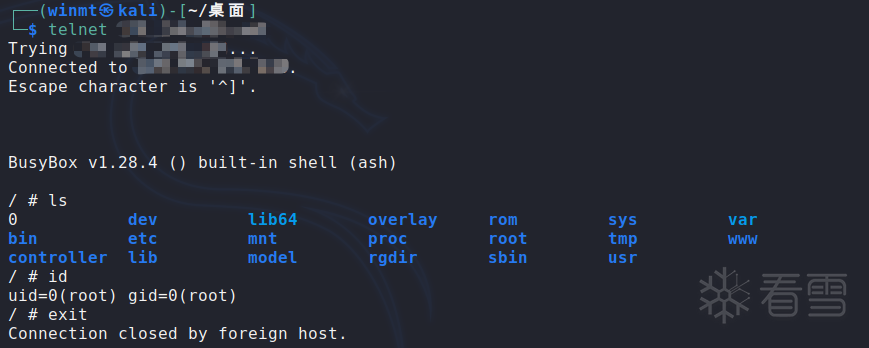## 仿真模拟

 1234567891011 `#!/bin/bash` `sudo qemu``-``system``-``mipsel \``    ``-``cpu ``74Kf` `\``    ``-``M malta \``    ``-``kernel vmlinux``-``3.2``.``0``-``4``-``4kc``-``malta \``    ``-``hda debian_squeeze_mipsel_standard.qcow2 \``    ``-``append ``"root=/dev/sda1 console=tty0"` `\``    ``-``net nic,macaddr``=``00``:``16``:``3e``:``00``:``00``:``01` `\``    ``-``net tap \``    ``-``nographic`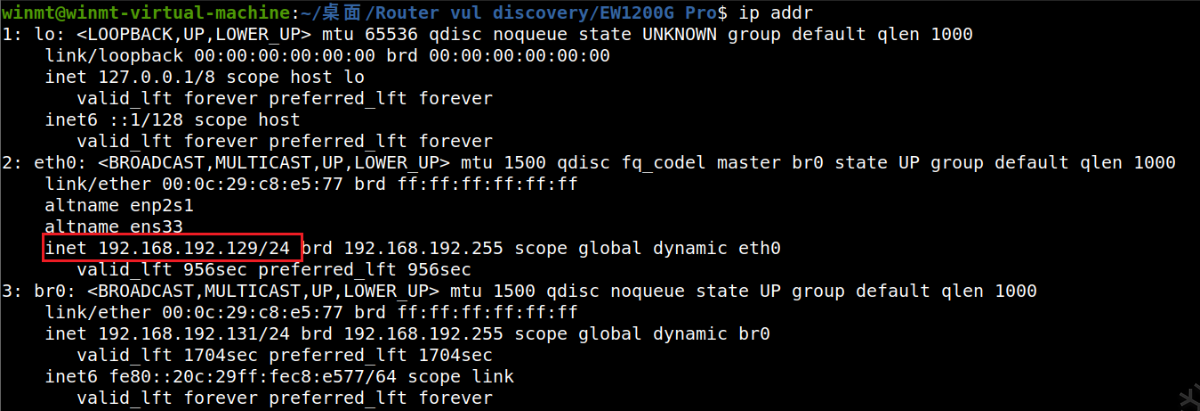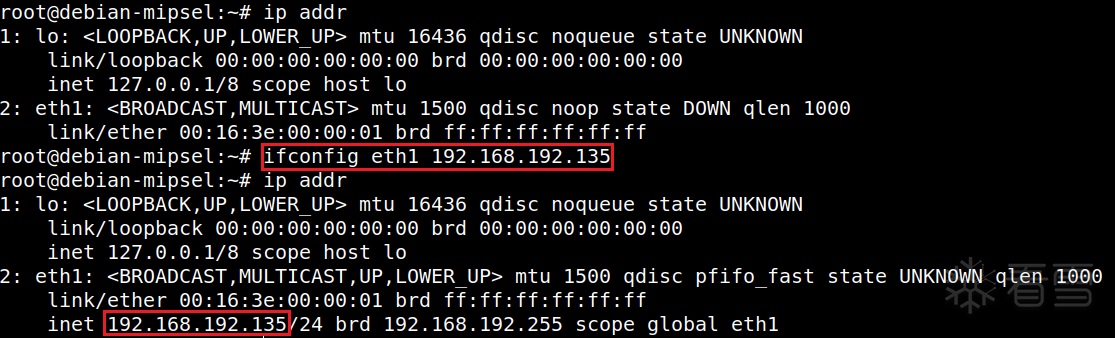12345 `cd rootfs``chmod ``-``R ``777` `.``/``mount ``-``-``bind ``/``proc proc``mount ``-``-``bind ``/``dev dev``chroot . ``/``bin``/``sh`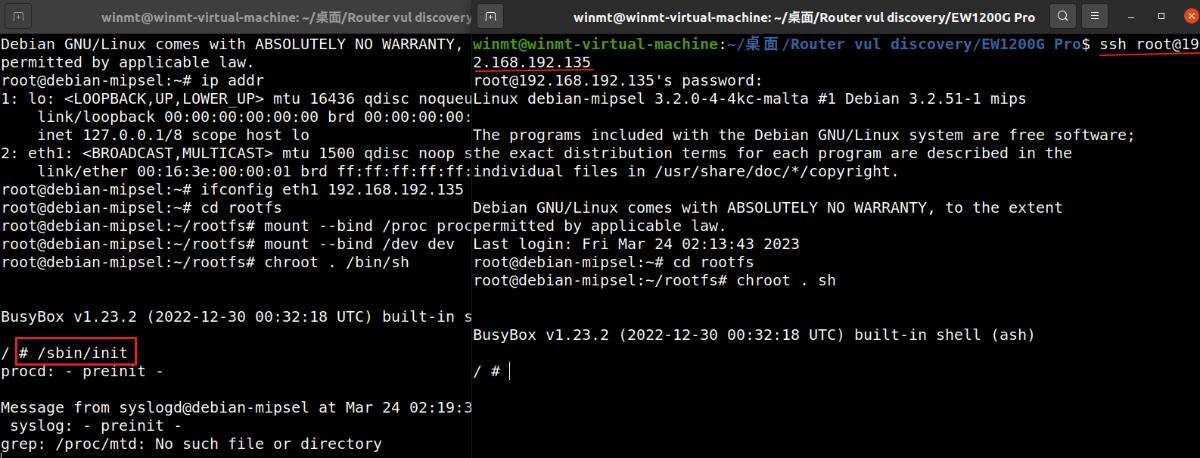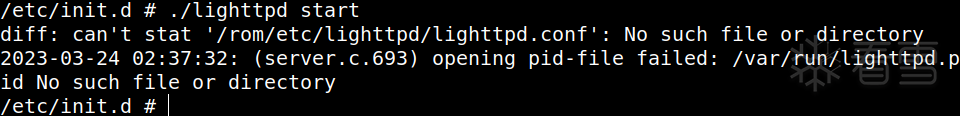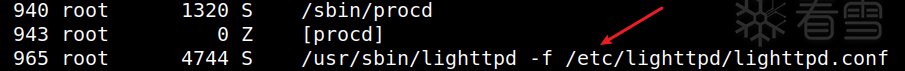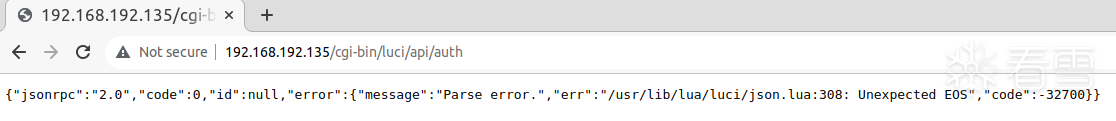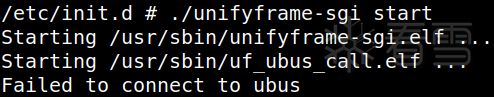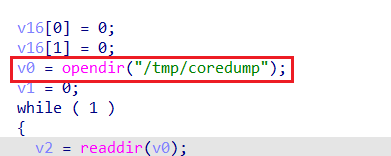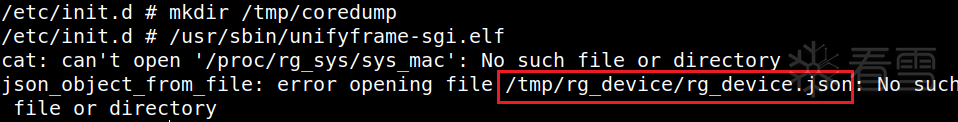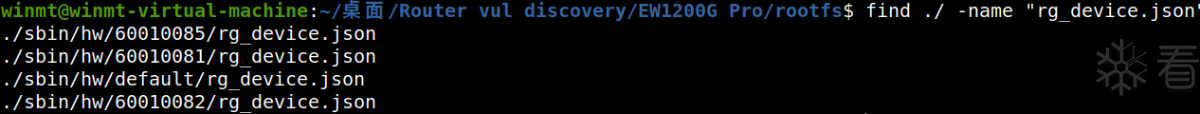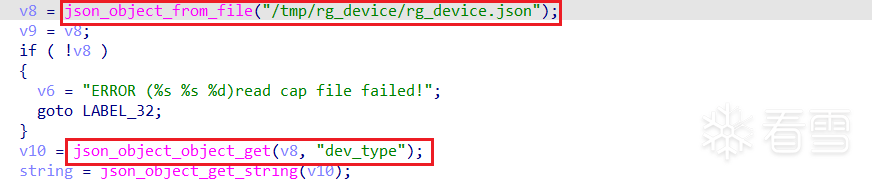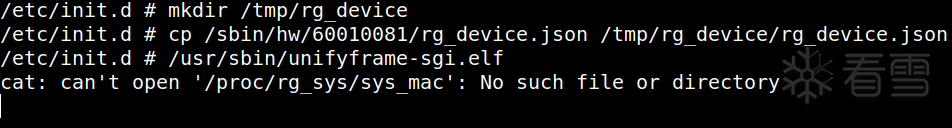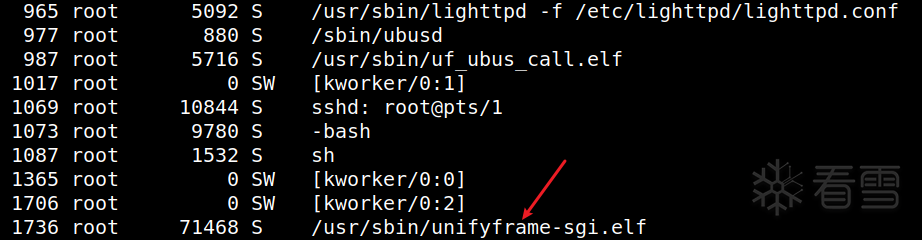## 补丁分析

### 补丁1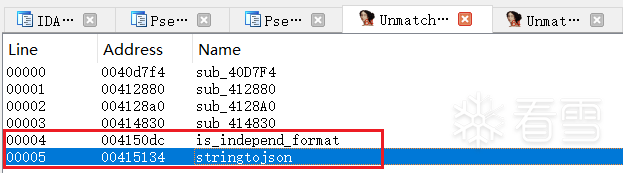`stringtojson`函数中，调用了`is_independ_format`函数，判断是否存在单引号和反引号。若不存在就返回，而返回的内容无法通过单引号闭合，也就无法执行任意命令。

 1234567891011121314151617 `__int64 __fastcall stringtojson(__int64 a1)``{``    ``...``    ``v2 ``=` `0x433000uLL``;``    ``v3 ``=` `0x433000uLL``;``    ``if` `( !a1 )``        ``goto LABEL_2;``    ``while` `( ``2` `)``    ``{``        ``v5 ``=` `(_BYTE ``*``)a1;``        ``if` `( !(``*``(unsigned __int8 (``*``*``)(void))(v2 ``+` `2968``))() ``/``/` `is_independ_format``            ``|| ... )``        ``{``        ``LABEL_2:``            ``v1 ``=` `0LL``;``            ``goto LABEL_3; ``/``/` `-``> ``return` `xxx;``        ``}`
 12345678 `bool` `__fastcall is_independ_format(const char ``*``a1)``{``  ``if` `( !a1 )``    ``return` `0LL``;``  ``if` `( strchr(a1, ``'`'``) )``    ``return` `1LL``;``  ``return` `strchr(a1, ``'\''``) !``=` `0LL``;``}`

 12345678910111213141516171819202122232425262728293031323334 `while` `( ``1` `)``{``    ``v13 ``=` `(unsigned __int8)``*``v11;``    ``if` `( !``*``v11 )``        ``break``;``    ``v15 ``=` `v11 ``+` `1``;``    ``if` `( v13 ``=``=` `'\''` `)``        ``goto LABEL_22;``    ``...``LABEL_22:``    ``if` `( v11 !``=` `(_BYTE ``*``)``1` `)``    ``{``        ``v13 ``=` `'u'``;``        ``v16 ``=` `*``(v11 ``-` `1``) !``=` `'\\'``;``        ``if` `( (unsigned __int64)v11 <``=` `v10 )``            ``goto LABEL_24; ``/``/` `mark``        ``goto LABEL_38;``    ``}``    ``goto LABEL_19;``    ``...``LABEL_24:``    ``if` `( !v16 )``        ``goto LABEL_40;``    ``v17 ``=` `(__int64 ``*``)v22;``    ``LOBYTE(v22[``1``]) ``=` `v13; ``/``/` `mark``LABEL_26:``    ``if` `( v13 ``=``=` `'u'` `)``    ``{``        ``(``*``(void (__fastcall ``*``*``)(__int64, const char ``*``, _QWORD))(v3 ``+` `3680``))( ``/``/` `sprintf``            ``(__int64)v17 ``+` `v16 ``+` `4``,``            ``"%02x"``,``            ``(unsigned __int8)``*``(v15 ``-` `1``)); ``/``/` `mark``        ``v18 ``=` `(unsigned ``int``)(v16 ``+` `6``);``    ``}`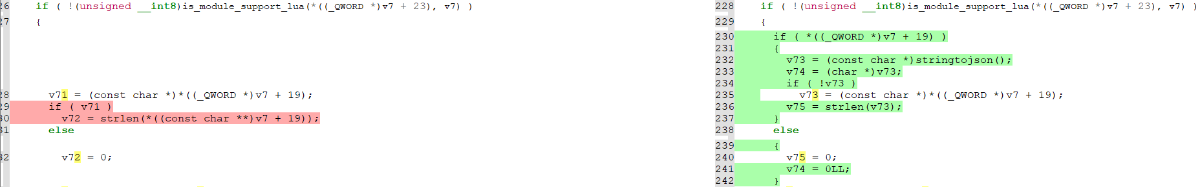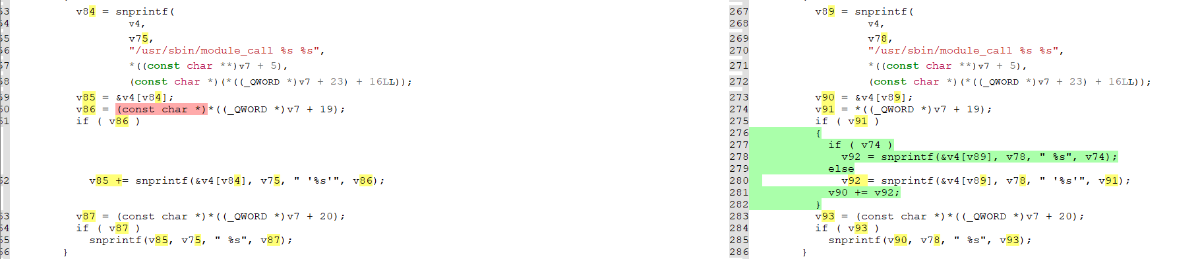1 `暂不公开`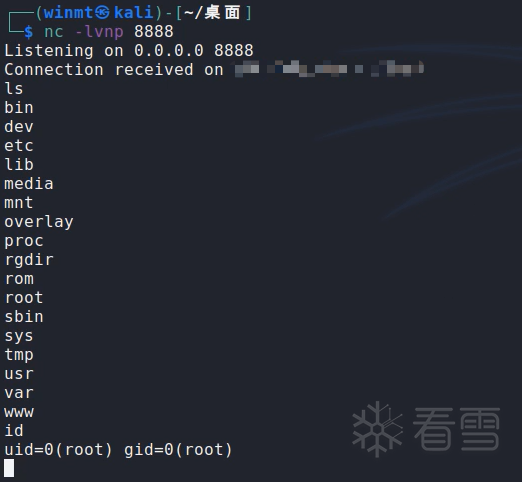### 补丁2

 123456789101112 `function merge(params)``    ``local cmd ``=` `require ``"luci.modules.cmd"``    ``local tool ``=` `require(``"luci.utils.tool"``)``    ``local _strParams ``=` `luci.json.encode(params)` `    ``if` `tool.includeXxs(_strParams) ``or` `tool.includeQuote(_strParams) then ``-``-` `过滤危险字符和单引号``        ``tool.eweblog(_strParams, ``"MERGE FAILED INVALID DATA"``)``        ``return` `'INVALID DATA'``    ``end` `    ``return` `cmd.devSta.``set``({device ``=` `"pc"``, module ``=` `"networkId_merge"``, data ``=` `params, async ``=` `true})``end`

 1234 `function includeXxs(``str``)``    ``local ngstr ``=` `"[\n`&\$;|]"``    ``return` `string.match(``str``, ngstr) ~``=` `nil``end`
 123 `function includeQuote(``str``)``    ``return` `string.match(``str``, ``"(['])"``) ~``=` `nil``end`

## 总结

[2023春季班]《安卓高级研修班(网课)》月薪两万班招生中～2023-5-26 22:48
2
02 2023-5-26 22:52
3
0
winmt我的神1 2023-5-26 23:54
4
02023-5-27 00:23
5
02023-5-27 14:38
6
0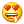太强了1 2023-5-27 22:45
7
02023-5-28 03:30
8
0
666膜拜一下2023-5-28 18:31
9
01 2023-5-28 19:58
10
11 2023-5-29 14:42
11
0
6662023-5-29 15:31
12
0
winmt我的神！2023-5-29 16:25
13
06 2023-5-31 16:44
14
02023-5-31 16:47
15
02023-6-1 09:05
16
16天前
17
0